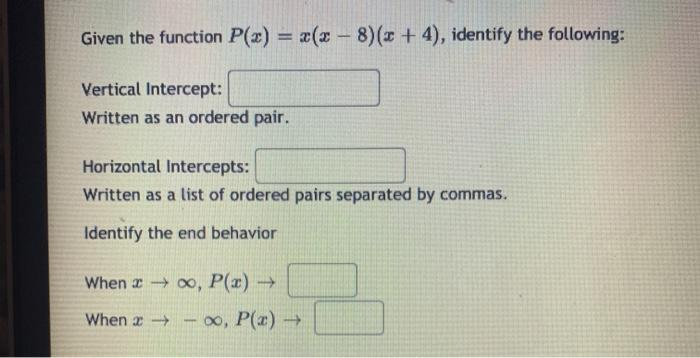### Create an Account

Home / Questions / Given the function P(x) = x(x-8)(3 + 4), identify the following: Vertical Intercept: Writt...

# Given the function P(x) = x(x-8)(3 + 4), identify the following: Vertical Intercept: Written as an ordered pair. Horizontal Intercepts: Written as a list of ordered pairs separated by commas. Identify

Given the function P(x) = x(x-8)(3 + 4), identify the following: Vertical Intercept: Written as an ordered pair. Horizontal Intercepts: Written as a list of ordered pairs separated by commas. Identify the end behavior When ro, P(x) > When x - , P(x)May 15 2021 View more View LessSubscribe To Get Solution